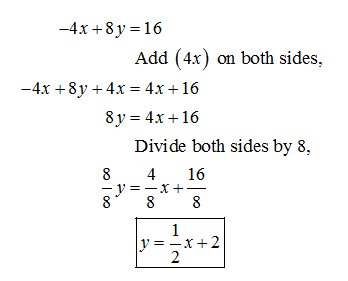Question
2 views

-4x+8y=16

Convert this to slope-intercept form.

check_circle

Step 1

Slope intercept form is y = mx +b, where, m = slope and b = y – intercept.

To convert the given equation in slope intercept form, simplify it....

### Want to see the full answer?

See Solution

#### Want to see this answer and more?

Solutions are written by subject experts who are available 24/7. Questions are typically answered within 1 hour.*

See Solution
*Response times may vary by subject and question.
Tagged in